# Maximum Likelihood Estimation¶

## Overview¶

In a previous lecture, we estimated the relationship between dependent and explanatory variables using linear regression.

But what if a linear relationship is not an appropriate assumption for our model?

One widely used alternative is maximum likelihood estimation, which involves specifying a class of distributions, indexed by unknown parameters, and then using the data to pin down these parameter values.

The benefit relative to linear regression is that it allows more flexibility in the probabilistic relationships between variables.

Here we illustrate maximum likelihood by replicating Daniel Treisman’s (2016) paper, Russia’s Billionaires, which connects the number of billionaires in a country to its economic characteristics.

The paper concludes that Russia has a higher number of billionaires than economic factors such as market size and tax rate predict.

We’ll require the following imports:

In :
import numpy as np
from numpy import exp
import matplotlib.pyplot as plt
%matplotlib inline
from scipy.special import factorial
import pandas as pd
from mpl_toolkits.mplot3d import Axes3D
import statsmodels.api as sm
from statsmodels.api import Poisson
from scipy import stats
from scipy.stats import norm
from statsmodels.iolib.summary2 import summary_col


### Prerequisites¶

We assume familiarity with basic probability and multivariate calculus.

## Set Up and Assumptions¶

Let’s consider the steps we need to go through in maximum likelihood estimation and how they pertain to this study.

### Flow of Ideas¶

The first step with maximum likelihood estimation is to choose the probability distribution believed to be generating the data.

More precisely, we need to make an assumption as to which parametric class of distributions is generating the data.

• e.g., the class of all normal distributions, or the class of all gamma distributions.

Each such class is a family of distributions indexed by a finite number of parameters.

• e.g., the class of normal distributions is a family of distributions indexed by its mean $\mu \in (-\infty, \infty)$ and standard deviation $\sigma \in (0, \infty)$.

We’ll let the data pick out a particular element of the class by pinning down the parameters.

The parameter estimates so produced will be called maximum likelihood estimates.

### Counting Billionaires¶

Treisman [Tre16] is interested in estimating the number of billionaires in different countries.

The number of billionaires is integer-valued.

Hence we consider distributions that take values only in the nonnegative integers.

(This is one reason least squares regression is not the best tool for the present problem, since the dependent variable in linear regression is not restricted to integer values)

One integer distribution is the Poisson distribution, the probability mass function (pmf) of which is

$$f(y) = \frac{\mu^{y}}{y!} e^{-\mu}, \qquad y = 0, 1, 2, \ldots, \infty$$

We can plot the Poisson distribution over $y$ for different values of $\mu$ as follows

In :
poisson_pmf = lambda y, μ: μ**y / factorial(y) * exp(-μ)
y_values = range(0, 25)

fig, ax = plt.subplots(figsize=(12, 8))

for μ in [1, 5, 10]:
distribution = []
for y_i in y_values:
distribution.append(poisson_pmf(y_i, μ))
ax.plot(y_values,
distribution,
label=f'$\mu$={μ}',
alpha=0.5,
marker='o',
markersize=8)

ax.grid()
ax.set_xlabel('$y$', fontsize=14)
ax.set_ylabel('$f(y \mid \mu)$', fontsize=14)
ax.axis(xmin=0, ymin=0)
ax.legend(fontsize=14)

plt.show()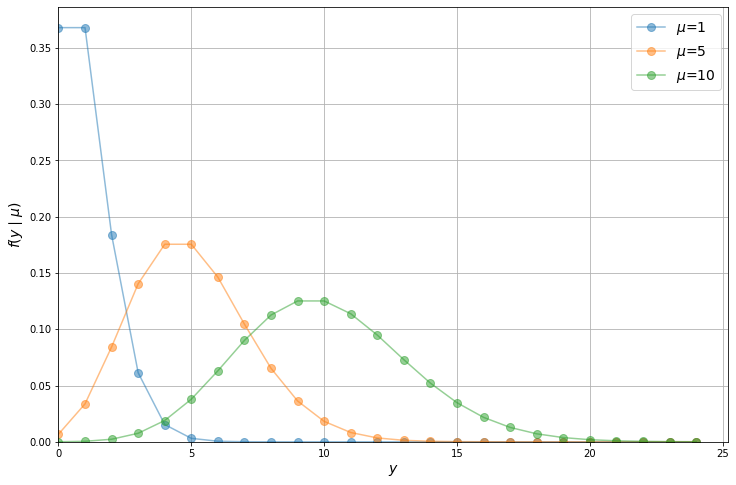Notice that the Poisson distribution begins to resemble a normal distribution as the mean of $y$ increases.

Let’s have a look at the distribution of the data we’ll be working with in this lecture.

Treisman’s main source of data is Forbes’ annual rankings of billionaires and their estimated net worth.

The dataset mle/fp.dta can be downloaded here or from its AER page.

In :
pd.options.display.max_columns = 10

# Load in data and view
df = pd.read_stata('https://github.com/QuantEcon/lecture-python/blob/master/source/_static/lecture_specific/mle/fp.dta?raw=true')
df.head()

Out:
country ccode year cyear numbil ... topint08 rintr noyrs roflaw nrrents
0 United States 2.0 1990.0 21990.0 NaN ... 39.799999 4.988405 20.0 1.61 NaN
1 United States 2.0 1991.0 21991.0 NaN ... 39.799999 4.988405 20.0 1.61 NaN
2 United States 2.0 1992.0 21992.0 NaN ... 39.799999 4.988405 20.0 1.61 NaN
3 United States 2.0 1993.0 21993.0 NaN ... 39.799999 4.988405 20.0 1.61 NaN
4 United States 2.0 1994.0 21994.0 NaN ... 39.799999 4.988405 20.0 1.61 NaN

5 rows × 36 columns

Using a histogram, we can view the distribution of the number of billionaires per country, numbil0, in 2008 (the United States is dropped for plotting purposes)

In :
numbil0_2008 = df[(df['year'] == 2008) & (
df['country'] != 'United States')].loc[:, 'numbil0']

plt.subplots(figsize=(12, 8))
plt.hist(numbil0_2008, bins=30)
plt.xlim(left=0)
plt.grid()
plt.xlabel('Number of billionaires in 2008')
plt.ylabel('Count')
plt.show()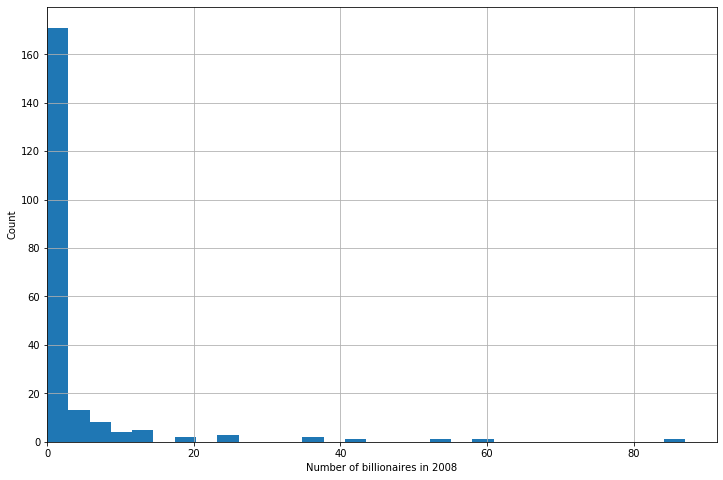From the histogram, it appears that the Poisson assumption is not unreasonable (albeit with a very low $\mu$ and some outliers).

## Conditional Distributions¶

In Treisman’s paper, the dependent variable — the number of billionaires $y_i$ in country $i$ — is modeled as a function of GDP per capita, population size, and years membership in GATT and WTO.

Hence, the distribution of $y_i$ needs to be conditioned on the vector of explanatory variables $\mathbf{x}_i$.

The standard formulation — the so-called poisson regression model — is as follows:

$$f(y_i \mid \mathbf{x}_i) = \frac{\mu_i^{y_i}}{y_i!} e^{-\mu_i}; \qquad y_i = 0, 1, 2, \ldots , \infty . \tag{1}$$

$$\text{where}\ \mu_i = \exp(\mathbf{x}_i' \boldsymbol{\beta}) = \exp(\beta_0 + \beta_1 x_{i1} + \ldots + \beta_k x_{ik})$$

To illustrate the idea that the distribution of $y_i$ depends on $\mathbf{x}_i$ let’s run a simple simulation.

We use our poisson_pmf function from above and arbitrary values for $\boldsymbol{\beta}$ and $\mathbf{x}_i$

In :
y_values = range(0, 20)

# Define a parameter vector with estimates
β = np.array([0.26, 0.18, 0.25, -0.1, -0.22])

# Create some observations X
datasets = [np.array([0, 1, 1, 1, 2]),
np.array([2, 3, 2, 4, 0]),
np.array([3, 4, 5, 3, 2]),
np.array([6, 5, 4, 4, 7])]

fig, ax = plt.subplots(figsize=(12, 8))

for X in datasets:
μ = exp(X @ β)
distribution = []
for y_i in y_values:
distribution.append(poisson_pmf(y_i, μ))
ax.plot(y_values,
distribution,
label=f'$\mu_i$={μ:.1}',
marker='o',
markersize=8,
alpha=0.5)

ax.grid()
ax.legend()
ax.set_xlabel('$y \mid x_i$')
ax.set_ylabel(r'$f(y \mid x_i; \beta )$')
ax.axis(xmin=0, ymin=0)
plt.show()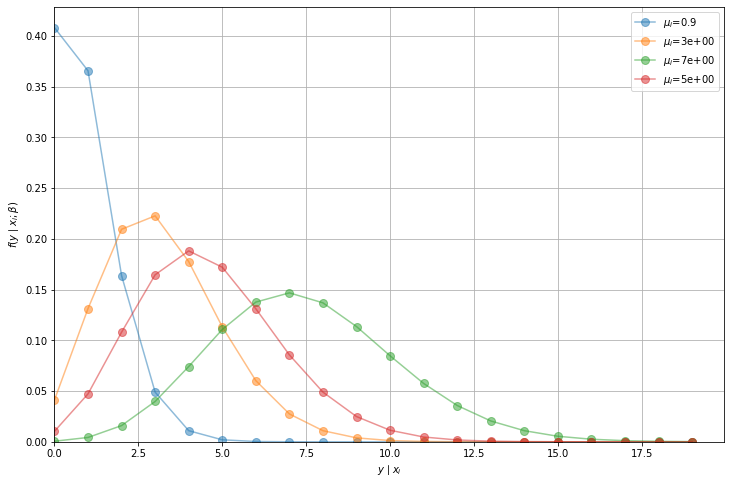We can see that the distribution of $y_i$ is conditional on $\mathbf{x}_i$ ($\mu_i$ is no longer constant).

## Maximum Likelihood Estimation¶

In our model for number of billionaires, the conditional distribution contains 4 ($k = 4$) parameters that we need to estimate.

We will label our entire parameter vector as $\boldsymbol{\beta}$ where

$$\boldsymbol{\beta} = \begin{bmatrix} \beta_0 \\ \beta_1 \\ \beta_2 \\ \beta_3 \end{bmatrix}$$

To estimate the model using MLE, we want to maximize the likelihood that our estimate $\hat{\boldsymbol{\beta}}$ is the true parameter $\boldsymbol{\beta}$.

Intuitively, we want to find the $\hat{\boldsymbol{\beta}}$ that best fits our data.

First, we need to construct the likelihood function $\mathcal{L}(\boldsymbol{\beta})$, which is similar to a joint probability density function.

Assume we have some data $y_i = \{y_1, y_2\}$ and $y_i \sim f(y_i)$.

If $y_1$ and $y_2$ are independent, the joint pmf of these data is $f(y_1, y_2) = f(y_1) \cdot f(y_2)$.

If $y_i$ follows a Poisson distribution with $\lambda = 7$, we can visualize the joint pmf like so

In :
def plot_joint_poisson(μ=7, y_n=20):
yi_values = np.arange(0, y_n, 1)

# Create coordinate points of X and Y
X, Y = np.meshgrid(yi_values, yi_values)

# Multiply distributions together
Z = poisson_pmf(X, μ) * poisson_pmf(Y, μ)

fig = plt.figure(figsize=(12, 8))
ax = fig.add_subplot(111, projection='3d')
ax.plot_surface(X, Y, Z.T, cmap='terrain', alpha=0.6)
ax.scatter(X, Y, Z.T, color='black', alpha=0.5, linewidths=1)
ax.set(xlabel='$y_1$', ylabel='$y_2$')
ax.set_zlabel('$f(y_1, y_2)$', labelpad=10)
plt.show()

plot_joint_poisson(μ=7, y_n=20)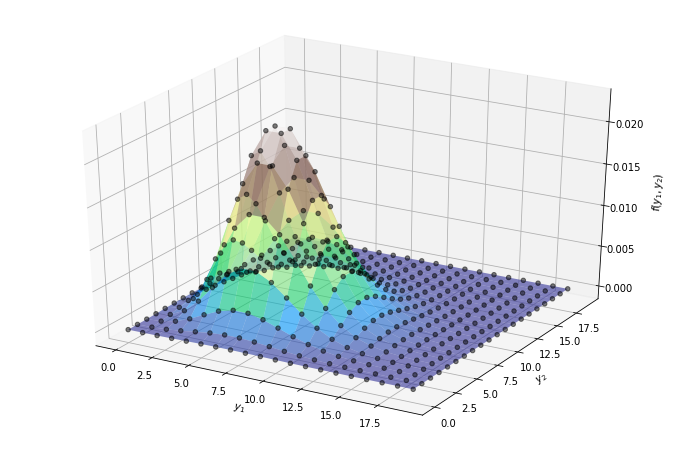Similarly, the joint pmf of our data (which is distributed as a conditional Poisson distribution) can be written as

$$f(y_1, y_2, \ldots, y_n \mid \mathbf{x}_1, \mathbf{x}_2, \ldots, \mathbf{x}_n; \boldsymbol{\beta}) = \prod_{i=1}^{n} \frac{\mu_i^{y_i}}{y_i!} e^{-\mu_i}$$

$y_i$ is conditional on both the values of $\mathbf{x}_i$ and the parameters $\boldsymbol{\beta}$.

The likelihood function is the same as the joint pmf, but treats the parameter $\boldsymbol{\beta}$ as a random variable and takes the observations $(y_i, \mathbf{x}_i)$ as given

$$\begin{split} \mathcal{L}(\beta \mid y_1, y_2, \ldots, y_n \ ; \ \mathbf{x}_1, \mathbf{x}_2, \ldots, \mathbf{x}_n) = & \prod_{i=1}^{n} \frac{\mu_i^{y_i}}{y_i!} e^{-\mu_i} \\ = & f(y_1, y_2, \ldots, y_n \mid \ \mathbf{x}_1, \mathbf{x}_2, \ldots, \mathbf{x}_n ; \beta) \end{split}$$

Now that we have our likelihood function, we want to find the $\hat{\boldsymbol{\beta}}$ that yields the maximum likelihood value

$$\underset{\boldsymbol{\beta}}{\max} \mathcal{L}(\boldsymbol{\beta})$$

In doing so it is generally easier to maximize the log-likelihood (consider differentiating $f(x) = x \exp(x)$ vs. $f(x) = \log(x) + x$).

Given that taking a logarithm is a monotone increasing transformation, a maximizer of the likelihood function will also be a maximizer of the log-likelihood function.

In our case the log-likelihood is

$$\begin{split} \log{ \mathcal{L}} (\boldsymbol{\beta}) = \ & \log \Big( f(y_1 ; \boldsymbol{\beta}) \cdot f(y_2 ; \boldsymbol{\beta}) \cdot \ldots \cdot f(y_n ; \boldsymbol{\beta}) \Big) \\ = & \sum_{i=1}^{n} \log{f(y_i ; \boldsymbol{\beta})} \\ = & \sum_{i=1}^{n} \log \Big( {\frac{\mu_i^{y_i}}{y_i!} e^{-\mu_i}} \Big) \\ = & \sum_{i=1}^{n} y_i \log{\mu_i} - \sum_{i=1}^{n} \mu_i - \sum_{i=1}^{n} \log y! \end{split}$$

The MLE of the Poisson to the Poisson for $\hat{\beta}$ can be obtained by solving

$$\underset{\beta}{\max} \Big( \sum_{i=1}^{n} y_i \log{\mu_i} - \sum_{i=1}^{n} \mu_i - \sum_{i=1}^{n} \log y! \Big)$$

However, no analytical solution exists to the above problem – to find the MLE we need to use numerical methods.

## MLE with Numerical Methods¶

Many distributions do not have nice, analytical solutions and therefore require numerical methods to solve for parameter estimates.

One such numerical method is the Newton-Raphson algorithm.

Our goal is to find the maximum likelihood estimate $\hat{\boldsymbol{\beta}}$.

At $\hat{\boldsymbol{\beta}}$, the first derivative of the log-likelihood function will be equal to 0.

Let’s illustrate this by supposing

$$\log \mathcal{L(\beta)} = - (\beta - 10) ^2 - 10$$
In :
β = np.linspace(1, 20)
logL = -(β - 10) ** 2 - 10
dlogL = -2 * β + 20

fig, (ax1, ax2) = plt.subplots(2, sharex=True, figsize=(12, 8))

ax1.plot(β, logL, lw=2)
ax2.plot(β, dlogL, lw=2)

ax1.set_ylabel(r'$log \mathcal{L(\beta)}$',
rotation=0,
labelpad=35,
fontsize=15)
ax2.set_ylabel(r'$\frac{dlog \mathcal{L(\beta)}}{d \beta}$ ',
rotation=0,
labelpad=35,
fontsize=19)
ax2.set_xlabel(r'$\beta$', fontsize=15)
ax1.grid(), ax2.grid()
plt.axhline(c='black')
plt.show()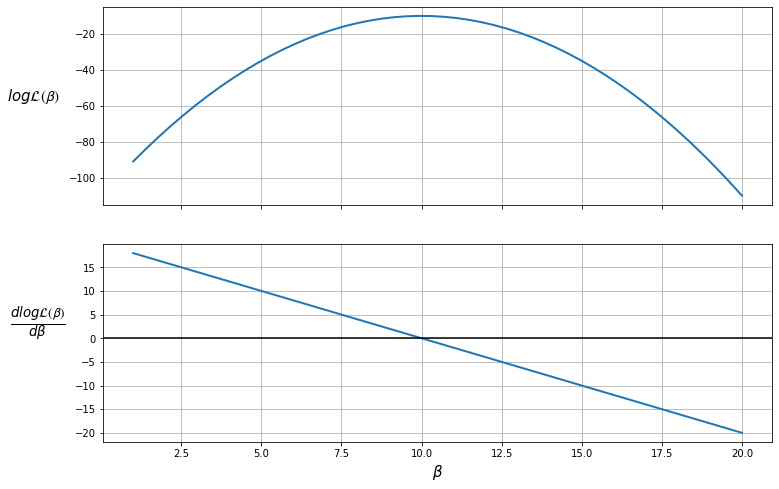The plot shows that the maximum likelihood value (the top plot) occurs when $\frac{d \log \mathcal{L(\boldsymbol{\beta})}}{d \boldsymbol{\beta}} = 0$ (the bottom plot).

Therefore, the likelihood is maximized when $\beta = 10$.

We can also ensure that this value is a maximum (as opposed to a minimum) by checking that the second derivative (slope of the bottom plot) is negative.

The Newton-Raphson algorithm finds a point where the first derivative is 0.

To use the algorithm, we take an initial guess at the maximum value, $\beta_0$ (the OLS parameter estimates might be a reasonable guess), then

1. Use the updating rule to iterate the algorithm
$$\boldsymbol{\beta}_{(k+1)} = \boldsymbol{\beta}_{(k)} - H^{-1}(\boldsymbol{\beta}_{(k)})G(\boldsymbol{\beta}_{(k)})$$ where:
\begin{aligned} G(\boldsymbol{\beta}_{(k)}) = \frac{d \log \mathcal{L(\boldsymbol{\beta}_{(k)})}}{d \boldsymbol{\beta}_{(k)}} \\ H(\boldsymbol{\beta}_{(k)}) = \frac{d^2 \log \mathcal{L(\boldsymbol{\beta}_{(k)})}}{d \boldsymbol{\beta}_{(k)}d \boldsymbol{\beta}'_{(k)}} \end{aligned}
2. Check whether $\boldsymbol{\beta}_{(k+1)} - \boldsymbol{\beta}_{(k)} < tol$
• If true, then stop iterating and set $\hat{\boldsymbol{\beta}} = \boldsymbol{\beta}_{(k+1)}$
• If false, then update $\boldsymbol{\beta}_{(k+1)}$

As can be seen from the updating equation, $\boldsymbol{\beta}_{(k+1)} = \boldsymbol{\beta}_{(k)}$ only when $G(\boldsymbol{\beta}_{(k)}) = 0$ ie. where the first derivative is equal to 0.

(In practice, we stop iterating when the difference is below a small tolerance threshold)

Let’s have a go at implementing the Newton-Raphson algorithm.

First, we’ll create a class called PoissonRegression so we can easily recompute the values of the log likelihood, gradient and Hessian for every iteration

In :
class PoissonRegression:

def __init__(self, y, X, β):
self.X = X
self.n, self.k = X.shape
# Reshape y as a n_by_1 column vector
self.y = y.reshape(self.n,1)
# Reshape β as a k_by_1 column vector
self.β = β.reshape(self.k,1)

def μ(self):
return np.exp(self.X @ self.β)

def logL(self):
y = self.y
μ = self.μ()
return np.sum(y * np.log(μ) - μ - np.log(factorial(y)))

def G(self):
y = self.y
μ = self.μ()
return X.T @ (y - μ)

def H(self):
X = self.X
μ = self.μ()
return -(X.T @ (μ * X))


Our function newton_raphson will take a PoissonRegression object that has an initial guess of the parameter vector $\boldsymbol{\beta}_0$.

The algorithm will update the parameter vector according to the updating rule, and recalculate the gradient and Hessian matrices at the new parameter estimates.

Iteration will end when either:

• The difference between the parameter and the updated parameter is below a tolerance level.
• The maximum number of iterations has been achieved (meaning convergence is not achieved).

So we can get an idea of what’s going on while the algorithm is running, an option display=True is added to print out values at each iteration.

In :
def newton_raphson(model, tol=1e-3, max_iter=1000, display=True):

i = 0
error = 100  # Initial error value

# Print header of output
if display:
header = f'{"Iteration_k":<13}{"Log-likelihood":<16}{"θ":<60}'
print(header)
print("-" * len(header))

# While loop runs while any value in error is greater
# than the tolerance until max iterations are reached
while np.any(error > tol) and i < max_iter:
H, G = model.H(), model.G()
β_new = model.β - (np.linalg.inv(H) @ G)
error = β_new - model.β
model.β = β_new

# Print iterations
if display:
β_list = [f'{t:.3}' for t in list(model.β.flatten())]
update = f'{i:<13}{model.logL():<16.8}{β_list}'
print(update)

i += 1

print(f'Number of iterations: {i}')
print(f'β_hat = {model.β.flatten()}')

# Return a flat array for β (instead of a k_by_1 column vector)
return model.β.flatten()


Let’s try out our algorithm with a small dataset of 5 observations and 3 variables in $\mathbf{X}$.

In :
X = np.array([[1, 2, 5],
[1, 1, 3],
[1, 4, 2],
[1, 5, 2],
[1, 3, 1]])

y = np.array([1, 0, 1, 1, 0])

# Take a guess at initial βs
init_β = np.array([0.1, 0.1, 0.1])

# Create an object with Poisson model values
poi = PoissonRegression(y, X, β=init_β)

# Use newton_raphson to find the MLE
β_hat = newton_raphson(poi, display=True)

Iteration_k  Log-likelihood  θ
-----------------------------------------------------------------------------------------
0            -4.3447622      ['-1.49', '0.265', '0.244']
1            -3.5742413      ['-3.38', '0.528', '0.474']
2            -3.3999526      ['-5.06', '0.782', '0.702']
3            -3.3788646      ['-5.92', '0.909', '0.82']
4            -3.3783559      ['-6.07', '0.933', '0.843']
5            -3.3783555      ['-6.08', '0.933', '0.843']
Number of iterations: 6
β_hat = [-6.07848205  0.93340226  0.84329625]


As this was a simple model with few observations, the algorithm achieved convergence in only 6 iterations.

You can see that with each iteration, the log-likelihood value increased.

Remember, our objective was to maximize the log-likelihood function, which the algorithm has worked to achieve.

Also, note that the increase in $\log \mathcal{L}(\boldsymbol{\beta}_{(k)})$ becomes smaller with each iteration.

This is because the gradient is approaching 0 as we reach the maximum, and therefore the numerator in our updating equation is becoming smaller.

The gradient vector should be close to 0 at $\hat{\boldsymbol{\beta}}$

In :
poi.G()

Out:
array([[-3.95169226e-07],
[-1.00114804e-06],
[-7.73114559e-07]])

The iterative process can be visualized in the following diagram, where the maximum is found at $\beta = 10$

In :
logL = lambda x: -(x - 10) ** 2 - 10

def find_tangent(β, a=0.01):
y1 = logL(β)
y2 = logL(β+a)
x = np.array([[β, 1], [β+a, 1]])
m, c = np.linalg.lstsq(x, np.array([y1, y2]), rcond=None)
return m, c

β = np.linspace(2, 18)
fig, ax = plt.subplots(figsize=(12, 8))
ax.plot(β, logL(β), lw=2, c='black')

for β in [7, 8.5, 9.5, 10]:
β_line = np.linspace(β-2, β+2)
m, c = find_tangent(β)
y = m * β_line + c
ax.plot(β_line, y, '-', c='purple', alpha=0.8)
ax.text(β+2.05, y[-1], f'$G({β}) = {abs(m):.0f}$', fontsize=12)
ax.vlines(β, -24, logL(β), linestyles='--', alpha=0.5)
ax.hlines(logL(β), 6, β, linestyles='--', alpha=0.5)

ax.set(ylim=(-24, -4), xlim=(6, 13))
ax.set_xlabel(r'$\beta$', fontsize=15)
ax.set_ylabel(r'$log \mathcal{L(\beta)}$',
rotation=0,
labelpad=25,
fontsize=15)
ax.grid(alpha=0.3)
plt.show()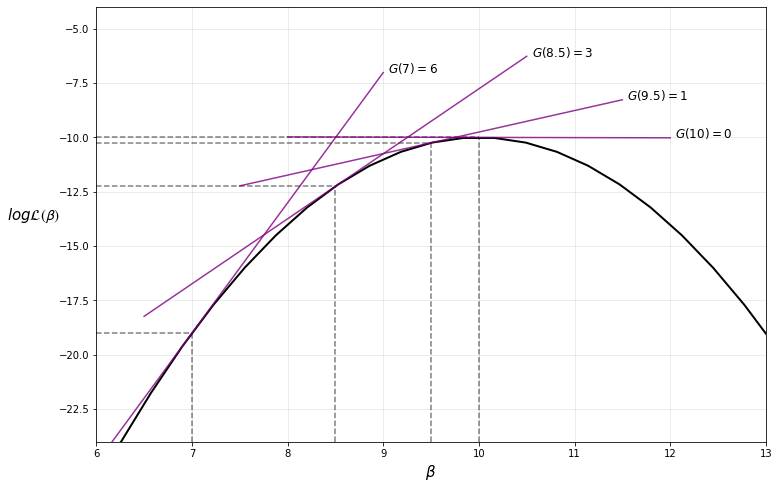Note that our implementation of the Newton-Raphson algorithm is rather basic — for more robust implementations see, for example, scipy.optimize.

## Maximum Likelihood Estimation with statsmodels¶

Now that we know what’s going on under the hood, we can apply MLE to an interesting application.

We’ll use the Poisson regression model in statsmodels to obtain a richer output with standard errors, test values, and more.

statsmodels uses the same algorithm as above to find the maximum likelihood estimates.

Before we begin, let’s re-estimate our simple model with statsmodels to confirm we obtain the same coefficients and log-likelihood value.

In :
X = np.array([[1, 2, 5],
[1, 1, 3],
[1, 4, 2],
[1, 5, 2],
[1, 3, 1]])

y = np.array([1, 0, 1, 1, 0])

stats_poisson = Poisson(y, X).fit()
print(stats_poisson.summary())

Optimization terminated successfully.
Current function value: 0.675671
Iterations 7
Poisson Regression Results
==============================================================================
Dep. Variable:                      y   No. Observations:                    5
Model:                        Poisson   Df Residuals:                        2
Method:                           MLE   Df Model:                            2
Date:                Tue, 09 Mar 2021   Pseudo R-squ.:                  0.2546
Time:                        11:20:54   Log-Likelihood:                -3.3784
converged:                       True   LL-Null:                       -4.5325
Covariance Type:            nonrobust   LLR p-value:                    0.3153
==============================================================================
coef    std err          z      P>|z|      [0.025      0.975]
------------------------------------------------------------------------------
const         -6.0785      5.279     -1.151      0.250     -16.425       4.268
x1             0.9334      0.829      1.126      0.260      -0.691       2.558
x2             0.8433      0.798      1.057      0.291      -0.720       2.407
==============================================================================


Now let’s replicate results from Daniel Treisman’s paper, Russia’s Billionaires, mentioned earlier in the lecture.

Treisman starts by estimating equation (1), where:

• $y_i$ is ${number\ of\ billionaires}_i$
• $x_{i1}$ is $\log{GDP\ per\ capita}_i$
• $x_{i2}$ is $\log{population}_i$
• $x_{i3}$ is ${years\ in\ GATT}_i$ – years membership in GATT and WTO (to proxy access to international markets)

The paper only considers the year 2008 for estimation.

We will set up our variables for estimation like so (you should have the data assigned to df from earlier in the lecture)

In :
# Keep only year 2008
df = df[df['year'] == 2008]

# Add a constant
df['const'] = 1

# Variable sets
reg1 = ['const', 'lngdppc', 'lnpop', 'gattwto08']
reg2 = ['const', 'lngdppc', 'lnpop',
'gattwto08', 'lnmcap08', 'rintr', 'topint08']
reg3 = ['const', 'lngdppc', 'lnpop', 'gattwto08', 'lnmcap08',
'rintr', 'topint08', 'nrrents', 'roflaw']


Then we can use the Poisson function from statsmodels to fit the model.

We’ll use robust standard errors as in the author’s paper

In :
# Specify model
poisson_reg = sm.Poisson(df[['numbil0']], df[reg1],
missing='drop').fit(cov_type='HC0')
print(poisson_reg.summary())

Optimization terminated successfully.
Current function value: 2.226090
Iterations 9
Poisson Regression Results
==============================================================================
Dep. Variable:                numbil0   No. Observations:                  197
Model:                        Poisson   Df Residuals:                      193
Method:                           MLE   Df Model:                            3
Date:                Tue, 09 Mar 2021   Pseudo R-squ.:                  0.8574
Time:                        11:20:54   Log-Likelihood:                -438.54
converged:                       True   LL-Null:                       -3074.7
Covariance Type:                  HC0   LLR p-value:                     0.000
==============================================================================
coef    std err          z      P>|z|      [0.025      0.975]
------------------------------------------------------------------------------
const        -29.0495      2.578    -11.268      0.000     -34.103     -23.997
lngdppc        1.0839      0.138      7.834      0.000       0.813       1.355
lnpop          1.1714      0.097     12.024      0.000       0.980       1.362
gattwto08      0.0060      0.007      0.868      0.386      -0.008       0.019
==============================================================================


Success! The algorithm was able to achieve convergence in 9 iterations.

Our output indicates that GDP per capita, population, and years of membership in the General Agreement on Tariffs and Trade (GATT) are positively related to the number of billionaires a country has, as expected.

Let’s also estimate the author’s more full-featured models and display them in a single table

In :
regs = [reg1, reg2, reg3]
reg_names = ['Model 1', 'Model 2', 'Model 3']
info_dict = {'Pseudo R-squared': lambda x: f"{x.prsquared:.2f}",
'No. observations': lambda x: f"{int(x.nobs):d}"}
regressor_order = ['const',
'lngdppc',
'lnpop',
'gattwto08',
'lnmcap08',
'rintr',
'topint08',
'nrrents',
'roflaw']
results = []

for reg in regs:
result = sm.Poisson(df[['numbil0']], df[reg],
missing='drop').fit(cov_type='HC0',
maxiter=100, disp=0)
results.append(result)

results_table = summary_col(results=results,
float_format='%0.3f',
stars=True,
model_names=reg_names,
info_dict=info_dict,
regressor_order=regressor_order)
results_table.add_title('Table 1 - Explaining the Number of Billionaires \
in 2008')
print(results_table)

Table 1 - Explaining the Number of Billionaires                         in 2008
=================================================
Model 1    Model 2    Model 3
-------------------------------------------------
const            -29.050*** -19.444*** -20.858***
(2.578)    (4.820)    (4.255)
lngdppc          1.084***   0.717***   0.737***
(0.138)    (0.244)    (0.233)
lnpop            1.171***   0.806***   0.929***
(0.097)    (0.213)    (0.195)
gattwto08        0.006      0.007      0.004
(0.007)    (0.006)    (0.006)
lnmcap08                    0.399**    0.286*
(0.172)    (0.167)
rintr                       -0.010     -0.009
(0.010)    (0.010)
topint08                    -0.051***  -0.058***
(0.011)    (0.012)
nrrents                                -0.005
(0.010)
roflaw                                 0.203
(0.372)
Pseudo R-squared 0.86       0.90       0.90
No. observations 197        131        131
=================================================
Standard errors in parentheses.
* p<.1, ** p<.05, ***p<.01


The output suggests that the frequency of billionaires is positively correlated with GDP per capita, population size, stock market capitalization, and negatively correlated with top marginal income tax rate.

To analyze our results by country, we can plot the difference between the predicted an actual values, then sort from highest to lowest and plot the first 15

In :
data = ['const', 'lngdppc', 'lnpop', 'gattwto08', 'lnmcap08', 'rintr',
'topint08', 'nrrents', 'roflaw', 'numbil0', 'country']
results_df = df[data].dropna()

# Use last model (model 3)
results_df['prediction'] = results[-1].predict()

# Calculate difference
results_df['difference'] = results_df['numbil0'] - results_df['prediction']

# Sort in descending order
results_df.sort_values('difference', ascending=False, inplace=True)

# Plot the first 15 data points
results_df[:15].plot('country', 'difference', kind='bar',
figsize=(12,8), legend=False)
plt.ylabel('Number of billionaires above predicted level')
plt.xlabel('Country')
plt.show()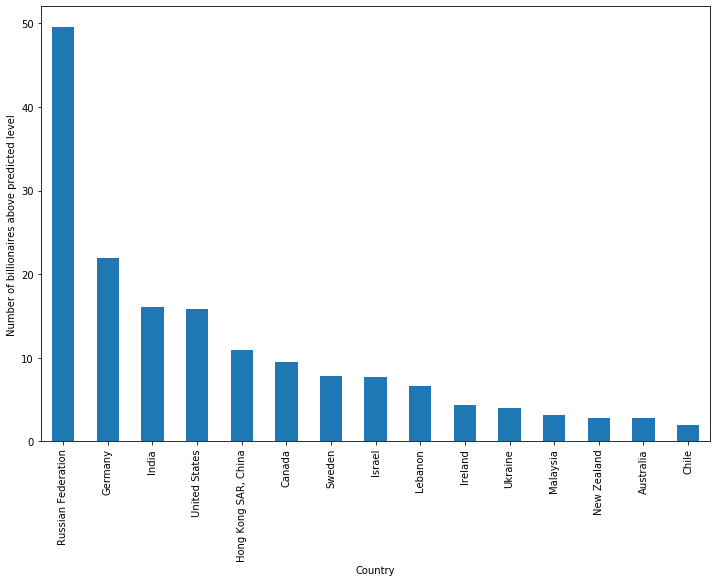As we can see, Russia has by far the highest number of billionaires in excess of what is predicted by the model (around 50 more than expected).

Treisman uses this empirical result to discuss possible reasons for Russia’s excess of billionaires, including the origination of wealth in Russia, the political climate, and the history of privatization in the years after the USSR.

## Summary¶

In this lecture, we used Maximum Likelihood Estimation to estimate the parameters of a Poisson model.

statsmodels contains other built-in likelihood models such as Probit and Logit.

For further flexibility, statsmodels provides a way to specify the distribution manually using the GenericLikelihoodModel class - an example notebook can be found here.

## Exercises¶

### Exercise 1¶

Suppose we wanted to estimate the probability of an event $y_i$ occurring, given some observations.

We could use a probit regression model, where the pmf of $y_i$ is

\begin{aligned} f(y_i; \boldsymbol{\beta}) = \mu_i^{y_i} (1-\mu_i)^{1-y_i}, \quad y_i = 0,1 \\ \text{where} \quad \mu_i = \Phi(\mathbf{x}_i' \boldsymbol{\beta}) \end{aligned}

$\Phi$ represents the cumulative normal distribution and constrains the predicted $y_i$ to be between 0 and 1 (as required for a probability).

$\boldsymbol{\beta}$ is a vector of coefficients.

Following the example in the lecture, write a class to represent the Probit model.

To begin, find the log-likelihood function and derive the gradient and Hessian.

The scipy module stats.norm contains the functions needed to compute the cmf and pmf of the normal distribution.

### Exercise 2¶

Use the following dataset and initial values of $\boldsymbol{\beta}$ to estimate the MLE with the Newton-Raphson algorithm developed earlier in the lecture

$$\mathbf{X} = \begin{bmatrix} 1 & 2 & 4 \\ 1 & 1 & 1 \\ 1 & 4 & 3 \\ 1 & 5 & 6 \\ 1 & 3 & 5 \end{bmatrix} \quad y = \begin{bmatrix} 1 \\ 0 \\ 1 \\ 1 \\ 0 \end{bmatrix} \quad \boldsymbol{\beta}_{(0)} = \begin{bmatrix} 0.1 \\ 0.1 \\ 0.1 \end{bmatrix}$$

Verify your results with statsmodels - you can import the Probit function with the following import statement

In :
from statsmodels.discrete.discrete_model import Probit


Note that the simple Newton-Raphson algorithm developed in this lecture is very sensitive to initial values, and therefore you may fail to achieve convergence with different starting values.

## Solutions¶

### Exercise 1¶

The log-likelihood can be written as

$$\log \mathcal{L} = \sum_{i=1}^n \big[ y_i \log \Phi(\mathbf{x}_i' \boldsymbol{\beta}) + (1 - y_i) \log (1 - \Phi(\mathbf{x}_i' \boldsymbol{\beta})) \big]$$

Using the fundamental theorem of calculus, the derivative of a cumulative probability distribution is its marginal distribution

$$\frac{ \partial} {\partial s} \Phi(s) = \phi(s)$$

where $\phi$ is the marginal normal distribution.

The gradient vector of the Probit model is

$$\frac {\partial \log \mathcal{L}} {\partial \boldsymbol{\beta}} = \sum_{i=1}^n \Big[ y_i \frac{\phi(\mathbf{x}'_i \boldsymbol{\beta})}{\Phi(\mathbf{x}'_i \boldsymbol{\beta)}} - (1 - y_i) \frac{\phi(\mathbf{x}'_i \boldsymbol{\beta)}}{1 - \Phi(\mathbf{x}'_i \boldsymbol{\beta)}} \Big] \mathbf{x}_i$$

The Hessian of the Probit model is

$$\frac {\partial^2 \log \mathcal{L}} {\partial \boldsymbol{\beta} \partial \boldsymbol{\beta}'} = -\sum_{i=1}^n \phi (\mathbf{x}_i' \boldsymbol{\beta}) \Big[ y_i \frac{ \phi (\mathbf{x}_i' \boldsymbol{\beta}) + \mathbf{x}_i' \boldsymbol{\beta} \Phi (\mathbf{x}_i' \boldsymbol{\beta}) } { [\Phi (\mathbf{x}_i' \boldsymbol{\beta})]^2 } + (1 - y_i) \frac{ \phi (\mathbf{x}_i' \boldsymbol{\beta}) - \mathbf{x}_i' \boldsymbol{\beta} (1 - \Phi (\mathbf{x}_i' \boldsymbol{\beta})) } { [1 - \Phi (\mathbf{x}_i' \boldsymbol{\beta})]^2 } \Big] \mathbf{x}_i \mathbf{x}_i'$$

Using these results, we can write a class for the Probit model as follows

In :
class ProbitRegression:

def __init__(self, y, X, β):
self.X, self.y, self.β = X, y, β
self.n, self.k = X.shape

def μ(self):
return norm.cdf(self.X @ self.β.T)

def ϕ(self):
return norm.pdf(self.X @ self.β.T)

def logL(self):
μ = self.μ()
return np.sum(y * np.log(μ) + (1 - y) * np.log(1 - μ))

def G(self):
μ = self.μ()
ϕ = self.ϕ()
return np.sum((X.T * y * ϕ / μ - X.T * (1 - y) * ϕ / (1 - μ)),
axis=1)

def H(self):
X = self.X
β = self.β
μ = self.μ()
ϕ = self.ϕ()
a = (ϕ + (X @ β.T) * μ) / μ**2
b = (ϕ - (X @ β.T) * (1 - μ)) / (1 - μ)**2
return -(ϕ * (y * a + (1 - y) * b) * X.T) @ X


### Exercise 2¶

In :
X = np.array([[1, 2, 4],
[1, 1, 1],
[1, 4, 3],
[1, 5, 6],
[1, 3, 5]])

y = np.array([1, 0, 1, 1, 0])

# Take a guess at initial βs
β = np.array([0.1, 0.1, 0.1])

# Create instance of Probit regression class
prob = ProbitRegression(y, X, β)

# Run Newton-Raphson algorithm
newton_raphson(prob)

Iteration_k  Log-likelihood  θ
-----------------------------------------------------------------------------------------
0            -2.3796884      ['-1.34', '0.775', '-0.157']
1            -2.3687526      ['-1.53', '0.775', '-0.0981']
2            -2.3687294      ['-1.55', '0.778', '-0.0971']
3            -2.3687294      ['-1.55', '0.778', '-0.0971']
Number of iterations: 4
β_hat = [-1.54625858  0.77778952 -0.09709757]

Out:
array([-1.54625858,  0.77778952, -0.09709757])
In :
# Use statsmodels to verify results

print(Probit(y, X).fit().summary())

Optimization terminated successfully.
Current function value: 0.473746
Iterations 6
Probit Regression Results
==============================================================================
Dep. Variable:                      y   No. Observations:                    5
Model:                         Probit   Df Residuals:                        2
Method:                           MLE   Df Model:                            2
Date:                Tue, 09 Mar 2021   Pseudo R-squ.:                  0.2961
Time:                        11:20:54   Log-Likelihood:                -2.3687
converged:                       True   LL-Null:                       -3.3651
Covariance Type:            nonrobust   LLR p-value:                    0.3692
==============================================================================
coef    std err          z      P>|z|      [0.025      0.975]
------------------------------------------------------------------------------
const         -1.5463      1.866     -0.829      0.407      -5.204       2.111
x1             0.7778      0.788      0.986      0.324      -0.768       2.323
x2            -0.0971      0.590     -0.165      0.869      -1.254       1.060
==============================================================================

• Share page